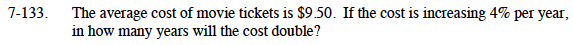Home > CCA2 > Chapter 7 > Lesson 7.2.2 > Problem7-133

7-133.

The average cost of movie tickets is \$9.50. If the cost is increasing 4% per year, in how many years will the cost double? Homework Help ✎Construct a representation of this scenario using the generic exponential equation:
y = abx

Solve for x (the number of years):
2(9.50) = 9.50(1.04)x

≈ 17.67 years